Reach Us+44-1474-556909
Approximation Solution to Solving Linear Volterra-Fredholm Integro-Differential Equations of the Second Kind by Using Bernstein Polynomials Method | OMICS International
Journal of Applied & Computational Mathematics
All submissions of the EM system will be redirected to Online Manuscript Submission System. Authors are requested to submit articles directly to Online Manuscript Submission System of respective journal.

# Approximation Solution to Solving Linear Volterra-Fredholm Integro-Differential Equations of the Second Kind by Using Bernstein Polynomials Method

Shahooth MK*, Ahmad RR, Din U-KS, Swidan W, Al-Husseini OK and Shahooth WK

Department of Mathematics, Faculty Science and Technology, National University of Malaysia, Malaysia

*Corresponding Author:
Shahooth MK
Department of Mathematics
Faculty Science and Technology
National University of Malaysia, Malaysia
Tel: +60389215555
E-mail: [email protected]

Received Date: February 15, 2016; Accepted Date: April 28, 2016; Published Date: May 04, 2016

Citation: Shahooth MK, Ahmad R, Salma U, Swidan W, Al-Husseini OK, et al. (2016) Approximation Solution to Solving Linear Volterra-Fredholm Integro-Differential Equations of the Second Kind by Using Bernstein Polynomials Method. J Appl Computat Math 5:298. doi:10.4172/2168-9679.1000298

Copyright: © 2016 Shahooth MK, et al. This is an open-access article distributed under the terms of the Creative Commons Attribution License, which permits unrestricted use, distribution, and reproduction in any medium, provided the original author and source are credited.

Visit for more related articles at Journal of Applied & Computational Mathematics

#### Abstract

The aim of this study is to present numerical method for solving the linear Volterra-Fredholm integro-differential equations of the second kind. This method is called the Bernestein polynomials method. This technique transforms the integro-differential equations to the system of algebraic equations. Some numerical results are presented to illustrate the efficiency and accuracy of this method.

#### Keywords

Bernstein polynomials; Volterra-Fredholm integro differential equations; Numerical solution; Mathematical tools

#### Introduction

Mathematical modelling of real-life problems usually results in functional equations, such an ordinary or partial differential equations, integral and integro-differential equations and stochastic equations. Many mathematical formulations of physical phenomena contain integro-differential an equation, these equations arises in many fields like fluid dynamics, biological models and chemical kinetics. In fact, integro-differential equations are usually difficult to solve analytically so it is required to obtain an efficient approximate or numerical solution [1,2].

There are several solution methods including Galerkin, collocation, Block Pulse Functions and direct method, for integro-differential equations have been studied [3-8].

In this study Bernstein polynomial method (BPM) is used to solve the linear Volterra-Fredholm integro-differential equation of the second kind: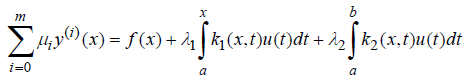(1)

with the initial condition y(i)(a)=yi, i=1,…., m

where a,b,λ12,yiare constants values, f(x),k1(x,t),k2(x,t) and μi,i=1,...,m with μi(x)≠0 are known functions that have derivatives on an interval axtb and y(x) is the unknown function which must be determined.

#### Bernstein Polynomials Method

Polynomials are incredibly useful mathematical tools as they are simple to define, can be calculated quickly on computer systems and represent a tremendous variety of functions. The Bernstein polynomials of degree-n are defined by :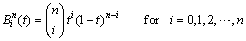(2)

where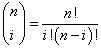,n is the degree of polynomials, i is the index of polynomials and t is the variable.

The exponents on the t term increase by one as i increase, and the exponents on the (1-t) term decrease by one as i increases.

The Bernstein polynomials of degree-n can be defined by blending together two Bernstein polynomials of degree-(n-1) that is, the kth nth-degree Bernstein polynomial can be written as :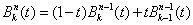(3)

Bernstein polynomials of degree-n can be written in terms of the power basis. This can be directly calculated using equation (2) and the Binomial theorem as follows :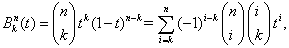where the Binomial theorem is used to expand (1-t)n-k.

The derivatives of the nth-degree Bernstein polynomials are polynomials of degree-(n-1).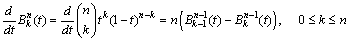(4)

#### A Matrix Representation for Bernstein Polynomials

In many applications, a matrix formulation for the Bernstein polynomials is useful. These are straight forward to develop if only looking at a linear combination in terms of dot products. Given a polynomial written as a linear combination of the Bernstein basis functions :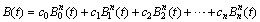(5)

The dot product of two vectors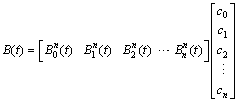(6)

which can be converted to the following form: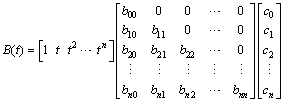(7)

where bnn are the coefficients of the power basis that are used to determine the respective Bernstein polynomials, we note that the matrix in this case is lower triangular. The matrix of derivatives of Bernstein polynomials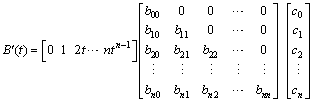(7a)

#### Solution for Volterra-Fredholm Integro-Differential Equations of the Second Kind

In this section, Bernstein polynomials method is proposed to find the approximate solution for Volterra-Fredholm integro-differential equations of the second kind.

Consider the Volterra-Fredholm integro-differential equations of the second kind in equation (1):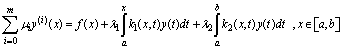(8)

Let y(x)=B(t) then,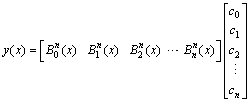(9)

Substituting (9) into equation (8), we get: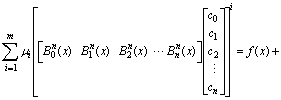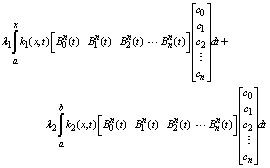(10)

Applying equation (7) into equation (10), we have: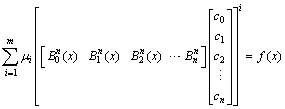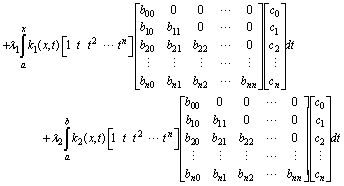(11)

Now to find all integration in equation (11). Then in order to determine C0,C1,…,Cn we need n equations. Now chose xi,i=1,2,3,…,n in the interval [a,b], which gives n equations. Solve the n equations by Gauss elimination to find the values of C0,C1,…,Cn. The following algorithmsummarizes the steps for finding the approximate solution for the second kind of linear Volterra-Fredholm integro-differential equations.

#### Algorithm (BPM)

Input: (f(x),k(x,t),y(x),a,b,x,λ12)

Output: Polynomials of degree n

Step 1: Choice n the degree of Bernstein polynomials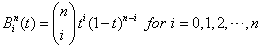Step 2: Put the Bernstein polynomials in linear Volterra-Fredholm integro-differential equations of second kind.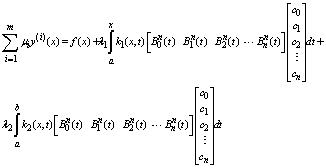Step 3:

Compute Volterra integral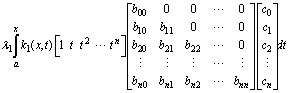Compute Fredholm integral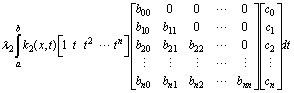Compute the term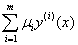Step 4:

Compute C0,C1,…,Cn, where xi,i=1,2,3,…,n, xi∈[a,b]

End.

#### Numerical Examples

In this section, two numerical examples are exhibited to illustrate the Bernstein polynomials method. The computations associated with the examples were performed using Matlab ver.2013a.

Example 1: Consider the linear Volterra-Fredholm integro-differential equation of the second kind .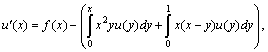with the initial condition u(0)=1, 0 ≤ x ≤ 1

Where f(x)=sin(x)–x2 cos(x)–x3 sin(x)+x2x2 sin(1)+x cos(1)+x sin(1)– x and the exact solution is u(x)=cos(x).

Tables 1 and 2 show that numerical results and the error respectively with the exact solution for Example 1 for n=1,4 and 7 by using BPM (Figure 1).

t yexact yapp, n=1 yapp, n=4 yapp, n=7
0 1.000000000000000 1.000000000000000 1.000000000000000 1.000000000000000
0.100000000000000 0.995004165278026 0.855760000000000 0.994277930000000 0.995005924500000
0.200000000000000 0.980066577841242 0.711520000000000 0.985024480000000 0.980071699200000
0.300000000000000 0.955336489125606 0.567280000000000 0.968301130000000 0.955343773900000
0.400000000000000 0.921060994002885 0.423040000000000 0.940052480000000 0.921068390400000
0.500000000000000 0.877582561890373 0.278800000000000 0.896106250000000 0.877589062500000
0.600000000000000 0.825335614909678 0.134560000000000 0.832173280000000 0.825341536000000
0.700000000000000 0.764842187284489 -0.009680000000000 0.743847530000000 0.764847740700000
0.800000000000000 0.696706709347165 -0.153920000000000 0.626606080000000 0.696710246400000
0.900000000000000 0.621609968270664 -0.298160000000000 0.475809130000000 0.621608734900000
1.000000000000000 0.540302305868140 -0.442400000000000 0.286700000000000 0.540300000000000

Table 1: Numerical results for Example 1 with exact solution by using BPM.

(yexact-yapp, n=1)2 (yexact-yapp, n=4)2 (yexact-yapp, n=7)2
0 0 0
0.019388937563974 0.000000527417679 0.000000000003095
0.072117264470242 0.000024580793816 0.000000000026228
0.150587838752492 0.000168081913002 0.000000000053068
0.248024910467622 0.000360676540379 0.000000000054707
0.358540556423998 0.000343127021183 0.000000000042258
0.477170950153844 0.000046753663887 0.000000000035059
0.599884618595948 0.000440775634493 0.000000000030840
0.723565798654787 0.004914098234869 0.000000000012511
0.845976794532619 0.021257884440428 0.000000000001521
0.965703821958559 0.064314129541638 0.000000000005317

Table 2: The errors for Example 1 by using BPM.

Example 2: Consider the linear Volterra-Fredholm integro differential equation of the second kind .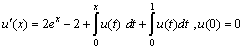and the exact solution is u(x)=xex (Figure 2).

#### Comparison with other Methods

In this part, the BPM was compared its performance with Repeated Trapezoidal method and Repeated Simpson’s 1/3 Method. A parameters here such as the degree of BPM and the error are considered as comparison. Throughout this manuscript, the convergence test with the proposed method is considered the last square error. We can notice that all the methods on the finite interval [a,b]. In BPM we proposed that we have a solution and we can develop it by increasing the degree of Bernstein polynomials method. Accordingly, the solution is convergence by increasing the number of the limits of Bernstein polynomials resulted from increasing the degree of Bernstein polynomials n, and the error decreases as results of that. As for Repeated Trapezoidal method and Repeated Simpson’s 1/3 Method, the solution is a result of Trapezoidal and Simpson’s 1/3 laws. Consequently, the error in this methods decrease in speed depending the h value which in its turn depends on the number of n points as mentioned earlier. Also, the accuracy of solution increases with the increase of n points number and the result will be a decrease at h value. Finally, the following table shows the error between these methods for example 1 (Table 3).

Points Repeated Trapezoidal
h=0.01
Repeated Simpson’s 1/3
h=0.01
Bernstein polynomials
yapp, n=7
0.100000000000000 0.000492742 0.000492400 0.000000000003095
0.200000000000000 0.000969158 0.000967886 0.000000000026228
0.300000000000000 0.00142909 0.00142649 0.000000000053068
0.400000000000000 0.00187288 0.00186873 0.000000000054707
0.500000000000000 0.00230186 0.00229612 0.000000000042258
0.600000000000000 0.00271897 0.00271179 0.000000000035059
0.700000000000000 0.00312960 0.00312126 0.000000000030840
0.800000000000000 0.00354248 0.00353340 0.000000000012511
0.900000000000000 0.00397088 0.00396158 0.000000000001521
1.000000000000000 0.00443427 0.00440738 0.000000000005317

Table 3: Comparison the error between Trapezoidal and Simpson’s 1/3 Methods with BPM for Example 1.

#### Conclusion

In the present study we have successfully used the proposed method to find an approximation solution for solving a second kind Volterra-Fredholm integro-differential equation. We noted from our results the approximation solution is close to the exact solution when we only used the degree of BPM is n=4 in example 1 and the error is small but still impossible to get satisfactory results with using this degree. When n=7 the result becomes so accuracy, so efficiency and the curve of an approximation solution is exactly over the curve of the exact solution. The figuring comes about additionally demonstrate that this strategy is so productive and it can be successfully use in the numerical arrangement of such sort mathematical statements. The integro differential equations are usually difficult to solve analytically so they are required to obtain an efficient approximated method. For this reason, the presented method have been proposed for approximated solution to the linear Volterra-Fredholm integro-differential equations of the second kind. From numerical examples it can be seen that the proposed numerical method is efficient and accurate to estimate the solution of these equations. Also we noted that when the degree of Bernstein polynomials is increasing the errors decrease to smaller values.

#### References

Select your language of interest to view the total content in your interested language

### Article Usage

• Total views: 10139
• [From(publication date):
May-2016 - Sep 20, 2019]
• Breakdown by view type
• HTML page views : 9786

## Post your commentCan't read the image? click here to refresh
###### Peer Reviewed Journals

Make the best use of Scientific Research and information from our 700 + peer reviewed, Open Access Journals

International Conferences 2019-20

Meet Inspiring Speakers and Experts at our 3000+ Global Annual Meetings

Top